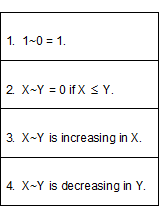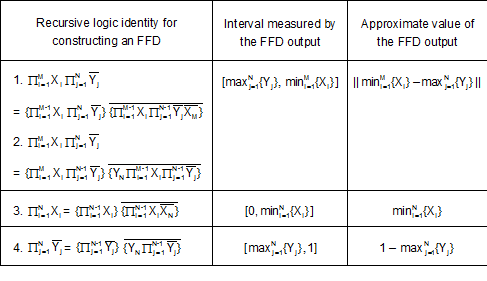## Fast Fuzzy Decoders

This is a brief summary of FFD architecture and its main property of fuzzy logic.  A more detailed description can be found in  in the Related Articles section.

A description of electronic FFDs is in preparation.

AND NOT Gates

Fast Fuzzy Decoders are composed of fuzzy AND NOT gates.  These gates each have two inputs, X and Y, and one output, X~Y, that satisfies the four properties in Table 1.Table 1.  Fuzzy AND NOT gate response properties.  A fuzzy AND NOT gate has two inputs, X and Y, and one output that satisfies the properties in the table.

Properties 1 and 2 imply that for binary inputs of 0 or 1, the output has the Boolean logic value of X AND NOT Y.  With the availability of a high input 1, fuzzy AND NOT gates are functionally complete, meaning for every possible Boolean logical proposition there is a way to define it in terms of the gates.

Fast Fuzzy Decoder Architecture

The Boolean logic identities in the first column of Table 2 show that every conjunction is logically equivalent to a single AND NOT conjunction A AND NOT B.  To make them clear, A and B are enclosed in braces.  The recursive and reductive identities show how any Boolean conjunction can be implemented entirely with Boolean AND NOT gates.Table 2.  FFD definitions and outputs.  The logic identities in the first column equate every conjunction to a conjunction A AND NOT B. The recursive and reductive identities show how decoders can be implemented with AND NOT gates.  The second column shows the interval measured by the corresponding FFD response.  The third column shows the approximate value of the response is the length of the interval.  The notation ||b – a|| stands for the length of the interval [a, b] if a < b, and 0 otherwise.

The number of possible ways of implementing a decoder according to the logic identities of Table 2 is quite large.  The architecture that minimizes the total cost of the number of gates, total connection length, and packing density is discussed in .  This architecture also has several other desirable properties that are discussed in the Advantages of FFDs section.

For 1 and 2 inputs, the FFD follows rather obviously from Table 2.  For 3 inputs, the most efficient FFD architecture has a hexagonal shape.  For n inputs, n > 3, the most efficient  architecture is defined recursively in terms of two FFDs, each with n-1 inputs.  If the n inputs are X1,…,Xn, the inputs to the first FFD are (NOT Xn)X1 ... (NOT Xn)Xn-1 and the inputs to the second are  Xn(NOTX1) ... Xn(NOTXn-1).

Main Property of Fast Fuzzy Decoders

A mathematical measure, usually designated by μ, is a broad generalization of length.  Here a function μ[a, b] is defined to be a measure of any interval [a, b] if (1) µ[0, 1] = 1; (2) μ[a, b] = 0 if a ≥ b; and (3) μ[a, b] < μ[c, d] if [a, b] is a proper subset of [c, d].  By this definition, the interval length max {0, b – a} is itself a measure of the interval [a, b].

If a decoder is constructed according to the identities of Table 2 and composed of fuzzy AND NOT gates defined by 1-4 above, then the decoder's output for the conjunction in the first column of Table 2 is a measure of the corresponding interval in the second column.  This property is called the FFD interval measure property.  If the component AND NOT gates are linear, the measure is the length of the interval, as shown in the third column of Table 2.  The FFD interval measure property is illustrated in the Interactive Color Vision Model’s depictions of the output cells.

The intuitive rationale for this interval measure truth value is that X1 ... XM (NOT Y1) ... (NOT YN) should have a high truth value if and only if all Xi have high truth values and all Yj have low truth values. A truth value that is a measure of the interval between the largest Yj and the smallest Xi is consistent with this view.

agape